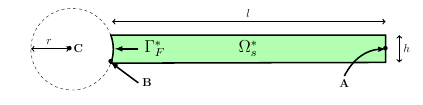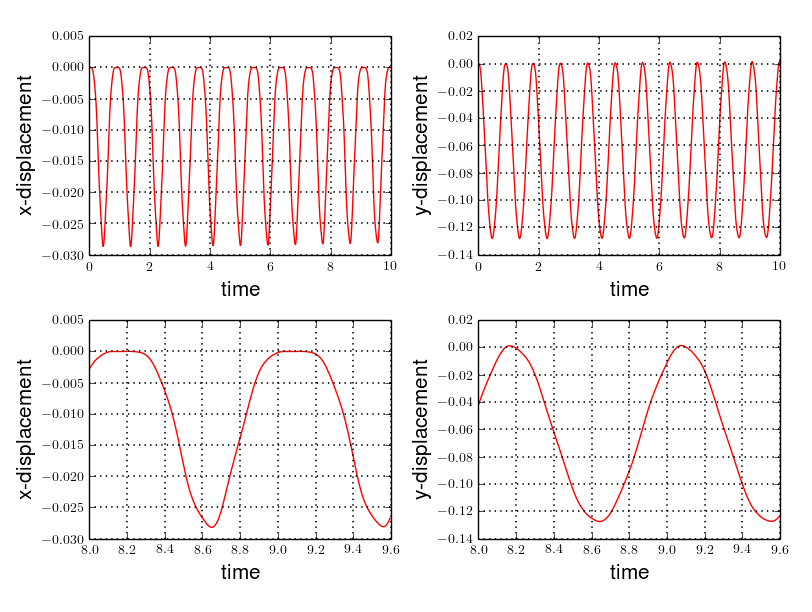# Turek Hron CSM Benchmark

In order to validate our fluid-structure interaction solver, we realize here a benchmark on the deformation of an elastic structure, initially proposed by [TurekHron].

Computer codes, used for the acquisition of results, are from [Chabannes].

 This benchmark is linked to the Turek Hron CFD and Turek Hron FSI benchmarks.

## 1. Problem Description

We consider a solid structure, composed of a hyperelastic bar, bound to one of his extremity $\Gamma_F^*$ to a rigid stationary circular structure. We denote $\Gamma_{L}^*=\partial\Omega_s^* \backslash \Gamma_F^*$ the other boundaries. The geometry can be represented as followsFigure 1. Geometry of the Turek & Hron CSM benchmark

$\Omega_s^*$ represent the initial domain, before any deformations. We denote $\Omega^t_s$ the domain obtained during the deformations application, at the time t.

By the Newton’s second law, we can describe the fundamental equation of the solid mechanic in a Lagrangian frame. Furthermore, we will suppose that the hyperelastic material follows a compressible Saint-Venant-Kirchhoff model. The Lamé coefficients, used in this model, are deduced from the Young’s modulus $E_s$ and the Poisson’s ratio $\nu_s$ of the material.

We observe, during this simulation, the displacement of $A$, on the $x$ and $y$ axis, when the elastic structure is subjected to its own weight, and compare our results to the reference ones given Turek and Hron.

In the first two cases, CSM1 and CSM2, we want to determine the steady state condition. To find it, a quasi-static algorithm is used, which increase at each step the gravity parameter.

For the third cases, CSM3, we realise a transient simulation, where we will observe the comportment of $A$ subjected to its weight during a given time span.

### 1.1. Boundary conditions

We set

• on $\Gamma_{F}^*$, a condition that imposes this boundary to be fixed : $\boldsymbol{\eta}_s=0$

• on $\Gamma_{L}^*$, a condition that lets these boundaries be free from constraints : $(\boldsymbol{F}_s\boldsymbol{\Sigma}_s)\boldsymbol{ n }^*_s=\boldsymbol{0}$

where $\boldsymbol{n}_s^*$ is the outer unit normal vector from $\partial \Omega_s^*$.

### 1.2. Initial conditions

The source term $\boldsymbol{f}_s$, chosen in order to set in motion the elastic structure, is define by

$\boldsymbol{f}_s=(0,-\rho^*_s g)$

where $g$ is a gravitational constant.

The reference document [TurekHron] doesn’t specify the time interval used to obtain their results. In our case, it’s chosen as $\lbrack0,10\rbrack$.

## 2. Inputs

The following table displays the various fixed and variables parameters of this test-case.

Table 1. Fixed and Variable Input Parameters
Name Description Nominal Value Units

$g$

gravitational constant

2

$m / s^2$

$l$

elastic structure length

$0.35$

$m$

$h$

elastic structure height

$0.02$

$m$

$r$

$0.05$

$m$

$C$

cylinder center coordinates

$(0.2,0.2)$

$m$

$A$

control point coordinates

$(0.2,0.2)$

$m$

$B$

point coordinates

$(0.15,0.2)$

$m$

$E_s$

Young’s modulus

$1.4\times 10^6$

$kg / ms^2$

$\nu_s$

Poisson’s ratio

$0.4$

dimensionless

$\rho^*_s$

density

$1000$

$kg/ m^3$

As for solvers we used, Newton’s method is chosen for the non-linear part and a direct method based on LU decomposition is selected for the linear part.

## 3. Outputs

As described before, we have

$\rho^*_s \frac{\partial^2\boldsymbol{\eta}_s}{\partial t^2} - \nabla \cdot (\boldsymbol{F}_s\boldsymbol{\Sigma}_s) = \boldsymbol{f}^t_s$

We search the displacement $\boldsymbol{\eta}_s$, on $\Omega_s^*$, which will satisfy this equation.

In particular, the displacement of the point $A$ is the one that interests us.

## 4. Discretization

To realize these tests, we made the choice to used Finite Elements Method, with Lagrangian elements of order $N$ to discretize space.

Newmark-beta method, presented into [Chabannes] papers, is the one we used for the time discretization. We used this method with $\gamma=0.5$ and $\beta=0.25$.

### 4.1. Solvers

Here are the different solvers ( linear and non-linear ) used during results acquisition.

 type gmres relative tolerance 1e-13 max iteration 1000 reuse preconditioner true
 relative tolerance 1e-8 steps tolerance 1e-8 max iteration 500 max iteration with reuse 10 reuse jacobian false reuse jacobian rebuild at first Newton step true
 relative tolerance 1e-5 max iteration 500 reuse preconditioner CSM1/CSM2 : false | CSM3 : true reuse preconditioner rebuild at first Newton step true
 type lu package mumps

## 5. Implementation

To realize the acquisition of the benchmark results, code files contained and using the Feel++ library will be used. Here is a quick look to the different location of them.

First at all, the main code can be found in

`    feelpp/applications/models/solid`

The configuration file for the CSM3 case, the only one we work on, is located at

`    feelpp/applications/models/solid/TurekHron`

The result files are then stored by default in

`    feel/applications/models/solid/TurekHron/csm3/"OrderDisp""Geometric_order"/"processor_used"`

Like that, for the CSM3 case executed on 8 processors, with a $P_1$ displacement approximation space and a geometric order of 1, the path is

`    feel/applications/models/solid/TurekHron/csm3/P1G1/np_8`

At least, to retrieve results that interested us for the benchmark and to generate graphs, we use a Python script located at

`    feelpp-benchmarking-book/CFD/Turek-Hron/postprocess_cfd.py`

## 6. Results

### 6.1. CSM1

 $N_{elt}$ $N_{dof}$ $x$ displacement $\lbrack\times 10^{-3}\rbrack$ $y$ displacement $\lbrack\times 10^{-3}\rbrack$ Reference [TurekHron] -7.187 -66.10 1061 4620 ($P_2$) -7.039 -65.32 4199 17540 ($P_2$) -7.047 -65.37 16495 67464 ($P_2$) -7.048 -65.37 1061 10112 ($P_3$) -7.046 -65.36 1906 17900 ($P_3$) -7.049 -65.37 1061 17726 ($P_4$) -7.048 -65.37

All the files used for this case can be found in this rep [ geo file, config file, json file ]

### 6.2. CSM2

 $N_{elt}$ $N_{dof}$ $x$ displacement $\lbrack\times 10^{-3}\rbrack$ $y$ displacement $\lbrack\times 10^{-3}\rbrack$ Reference [TurekHron] -0.4690 -16.97 1061 4620 ($P_2$) -0.459 -16.77 4201 17548 ($P_2$) -0.459 -16.77 16495 67464 ($P_2$) -0.459 -16.78 1061 10112 ($P_3$) -0.4594 -16.78 16475 150500 ($P_3$) -0.460 -16.78 1061 17726 ($P_4$) -0.460 -16.78

All the files used for this case can be found in this rep [geo file, config file, json file].

### 6.3. CSM3

The results of the CSM3 benchmark are detailed below.

 $\Delta t$ $N_{elt}$ $N_{dof}$ $x$ displacement $\lbrack\times 10^{-3}\rbrack$ $y$ displacement $\lbrack\times 10^{-3}\rbrack$ / Reference [TurekHron] −14.305 ± 14.305 [1.0995] −63.607 ± 65.160 [1.0995]
 0.02 4199 17536($P_2$) -14.585 ± 14.590 [1.0953] -63.981 ± 65.521 [1.0930] 4199 38900($P_3$) -14.589 ± 14.594 [1.0953] -63.998 ± 65.522 [1.0930] 1043 17536($P_4$) -14.591 ± 14.596 [1.0953] -64.009 ± 65.521 [1.0930] 4199 68662($P_4$) -14.590 ± 14.595 [1.0953] -64.003 ± 65.522 [1.0930]
 0.01 4199 17536($P_2$) -14.636 ± 14.640 [1.0969] -63.937 ± 65.761 [1.0945] 4199 38900($P_3$) -14.642 ± 14.646 [1.0969] -63.949 ± 65.771 [1.0945] 1043 17536($P_4$) -14.645 ± 14.649 [1.0961] -63.955 ± 65.778 [1.0945] 4199 68662($P_4$) -14.627 ± 14.629 [1.0947] -63.916 ± 65.739 [1.0947]
 0.005 4199 17536($P_2$) -14.645 ± 14.645 [1.0966] -64.083 ± 65.521 [1.0951] 4199 38900($P_3$) -14.649 ± 14.650 [1.0966] -64.092 ± 65.637 [1.0951] 1043 17536($P_4$) -14.652 ± 14.653 [1.0966] -64.099 ± 65.645 [1.0951] 4199 68662($P_4$) -14.650 ± 14.651 [1.0966] -64.095 ± 65.640 [1.0951]Figure 2. x and y displacements

All the files used for this case can be found in this rep [ geo file, config file, json file ]

### 6.4. Conclusion

To obtain these data, we used several different mesh refinements and different polynomial approximations for the displacement on the time interval $\lbrack 0,10 \rbrack$.

Our results are pretty similar to those from Turek and Hron, despite a small gap. This gap can be caused by the difference between our time interval and the one used for the reference acquisitions.

## 7. Bibliography

References for this benchmark
• [TurekHron] S. Turek and J. Hron, Proposal for numerical benchmarking of fluid-structure interaction between an elastic object and laminar incompressible flow, Lecture Notes in Computational Science and Engineering, 2006.

• [Chabannes] Vincent Chabannes, Vers la simulation numérique des écoulements sanguins, Équations aux dérivées partielles [math.AP], Université de Grenoble, 2013.